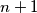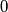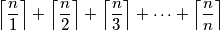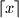### IMO Shortlist 2018 problem C3

Kvaliteta:
Avg: 0,0
Težina:
Avg: 7,0

Let$n$ be a given positive integer. Sisyphus performs a sequence of turns on a board consisting of$n + 1$ squares in a row, numbered$0$ to$n$ from left to right. Initially,$n$ stones are put into square$0$, and the other squares are empty. At every turn, Sisyphus chooses any nonempty square, say with$k$ stones, takes one of these stones and moves it to the right by at most$k$ squares (the stone should say within the board). Sisyphus' aim is to move all$n$ stones to square$n$. Prove that Sisyphus cannot reach the aim in less thanturns. (As usual,$\lceil x \rceil$ stands for the least integer not smaller than$x$. )

Izvor: https://www.imo-official.org/problems/IMO2018SL.pdf